Courses

# Thermodynamics 3 MCQ

## 20 Questions MCQ Test Mock Test Series for SSC JE Mechanical Engineering | Thermodynamics 3 MCQ

Description
This mock test of Thermodynamics 3 MCQ for Mechanical Engineering helps you for every Mechanical Engineering entrance exam. This contains 20 Multiple Choice Questions for Mechanical Engineering Thermodynamics 3 MCQ (mcq) to study with solutions a complete question bank. The solved questions answers in this Thermodynamics 3 MCQ quiz give you a good mix of easy questions and tough questions. Mechanical Engineering students definitely take this Thermodynamics 3 MCQ exercise for a better result in the exam. You can find other Thermodynamics 3 MCQ extra questions, long questions & short questions for Mechanical Engineering on EduRev as well by searching above.
QUESTION: 1

Solution:
QUESTION: 2

### At critical temperature the _______.

Solution:

The critical temperature is the highest temperature at which vapour and liquid phases can coexist in equilibrium. For gases, the critical temperature is the one above which a gas cannot be liquefied regardless of the pressure applied. Also, note that dryness fraction is not defined at the critical point

QUESTION: 3

### If the entropy of the universe decreases. What this depicts about the nature of the process?

Solution:

The universe is formed by system and surrounding and considered as an isolated system.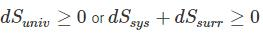i) The energy of the world (universe) is constant.

ii) The entropy of the world tends towards a maximum.

iii) The entropy increase of an isolated system is a measure of the extent of irreversibility of the process undergone by the system.

In actual practice the reversible isentropic process never really occurs, it is only an ideal process. In actual practice whenever there is a change in the state of the system the entropy of the system increases. The entropy of the universe increases because energy never flows uphill spontaneously.

If in any process, if the entropy of the universe decreases, then the process will be an impossible process.

QUESTION: 4

Which of the following properties are NOT sufficient to determine the properties of a vapour?

1. Temperature
2. Pressure
3. Dryness fraction
4. Specific volume
Solution:

In properties of vapour, we require at least two independent properties to determine the state of the vapour. Both pressure and temperature are dependent properties so, if we know only pressure and temperature, we can’t determine the properties of vapour.

QUESTION: 5

If the ratio of the lower to the higher absolute temperature is 7/8, then what will be the COP of the Carnot refrigerator?

Solution:

The Carnot COP of a refrigerator working between the temperature limit of TH and TL is given as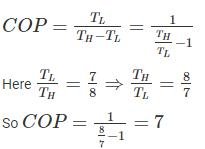Kindly note that the COP of Carnot heat pump or refrigerator depends only on the upper and lower temperature limits and COP is independent of the working fluid.

QUESTION: 6

One kg of air (R=287 J/kg-K) goes through an irreversible process between two equilibrium state 1 (30°C, 1.2 m3) and state 2 (30°C, 0.8 m3). What is the change in entropy (in J/kg-K)?

Solution:

Change of entropy in a reversible process: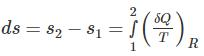Entropy changes for a closed system: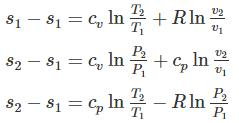For constant volume: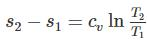For constant pressure: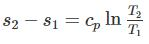For constant temperature process: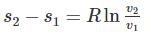dQ = 0 ⇒ dS = 0

Calculation:

Here T1 = T2

So the process is isothermal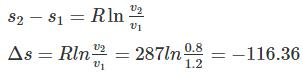QUESTION: 7

In a surrounding, the amount of irreversibility of a process undergone by a system is determined by ________.

Solution:

The universe is formed by system and surrounding and considered as an isolated system. The entropy of the universe always tends towards a maximum. The entropy increase of an isolated system is a measure of the extent of irreversibility of the process undergone by the system.

QUESTION: 8

Clausius’ statement and Kelvin - Planck’s statement are ________.

Solution:

There are two statements of second law of thermodynamics.

Clausius statement: No device can operate on a cycle and produce effect that is solely the heat transfer from a lower-temperature body to a higher-temperature body

Kelvin Plank statement: It is impossible for any device that operates on a cycle to receive heat from a single reservoir and produce a net amount of work

Equivalence of two statements: If some device violates one statement, it also violates the other statement, and vice versa.

QUESTION: 9

A Carnot engine operates between temperature 1000 K and 400 K. The heat rejected by the first Carnot engine is used by the second Carnot engine, whose sink temperature is 200 K. If the net heat absorbed by the first Carnot engine is 200 MJ. What is the heat rejected (in MJ) by the second Carnot engine?

Solution:
QUESTION: 10

In a general compression process, 2 kJ of mechanical work is supplied to 4 kg of fluid and 800 J of heat is rejected to the cooling jacket. The change in specific internal energy would be

Solution:

From the first law of thermodynamics

dQ = dU + dW

dQ = -800 J (As the heat is rejected from the system)

dW = -2000 J (as work is supplied to system)

Change in internal energy = dQ – dW = -800 – (-2000) = 1200 J

Change in specific internal energy = 1200/4 = 300 J/kg  (mass is 4 kg)

QUESTION: 11

Which of the below stated are properties of a PMM-2?

1. When the net work is equal to the heat absorbed and work efficiency is 100%.
2. Heat is exchanged from one heat reservoir only.
3. It violates Kelvin-Planck statement.
4. It is a hypothetical machine
Solution:

Kelvin – Planck statement states “it is impossible to construct an engine, which is operating in a cycle produces no other effect except to external heat from a single reservoir and do equivalent amount of work.

A PMM II is the heat engine which produces the work equal to the heat supplied. Thus, it violates the second law of thermodynamics and It is a hypothetical machine.

On the other hand, Perpetual motion machine of type-I violates the first law of thermodynamics.

QUESTION: 12

A gas having a negative Joule-Thomson coefficient (μJ < 0), when throttled, will

Solution: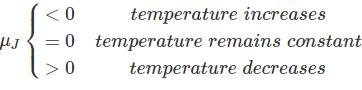QUESTION: 13

According to which law, all perfect gases change in volume by (1/273)th of their original volume at 0°C for every 1°C change in temperature when pressure remains constant

Solution:

temperature, when the absolute pressure remains constant." Mathematically,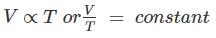Or “All perfect gases change in volume by 1 / 273 th of its original volume at 0° C for every 1° C change in temperature, when the pressure remains constant."

Boyles law: The absolute pressure of a given mass of a perfect gas varies inversely as its volume, when the temperature remains constant." Mathematically,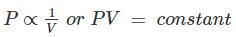QUESTION: 14

For a single component three phase mixture, the number of independent variable properties are

Solution:

As per Gibb’s phase rule,

F = C – P + 2

Where F = Degree of freedom

C = Number of chemical components

P = No of Phases

F = 1 – 3 + 2 = 0

QUESTION: 15

Three states of matter are distinguished with respect to molecules by the ______.

Solution:

Matter around us exists in three different states – solid, liquid and gas. They are distinguished by their intermolecular attraction force, molecular arrangement and the mobility of the system.

Particles in a:

• Gas vibrate and move freely at high speeds.
• Liquid vibrate, move about, and slide past each other.
• Solid vibrate (jiggle) but generally do not move from place to place.
QUESTION: 16

The triple point on a P - V diagram is ________.

Solution:
QUESTION: 17

An automobile heats up while lying in a parking lot on a sunny day. The process can be assumed to be

Solution:

The process of heating up of an automobile can be assumed as isobaric. Because the pressure inside the car is constant. So out of given options the option b is correct.

QUESTION: 18

While working between temperatures 150 K and 300 K, the entropy change experienced by the Carnot engine during heat addition is 1 kJ/K, the work produced (kJ) by the engine is ________.

Solution:
QUESTION: 19

In free expansion process

Solution:

No heat interaction takes place

Hence dQ = 0

From first law

dQ = du + dW

du = 0

QUESTION: 20

There is fixed mass and energy in

Solution:

Closed System: There is no mass transfer across the system boundary but energy transfer takes place into or out of the system.

Open System: In this type of system both mass and energy transfer takes place across the boundary of system.

Isolated System: In this type of system neither mass nor energy crosses the boundary of the system. So it is a system with fixed mass and fixed energy.